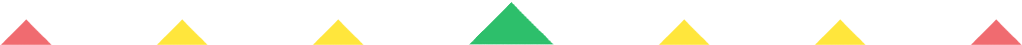Player 1
vs
Player 2
0:00
Opponent history
x
xCorrect
0
Wrong
0
Correct!
Wrong!
Help Sheet for

###• Half of 2 = 1
• Half of 4 = 2
• Half of 6 = 3
• Half of 8 = 4
• Half of 10 = 5
• Half of 12 = 6
• Half of 14 = 7
• Half of 16 = 8
• Half of 18 = 9
• Half of 20 = 10
• Half of 22 = 11
• Half of 24 = 12